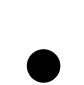Bag Of Cows

# Imperial Flag of Leachonia

Here is some code you can use

``````
from turtle import *
import random

# set speed to max, and hide the turtle
speed(0)
ht()

# set the size of a pixel
PIXEL_SIZE=30

# subroutine to draw a pixel in a colour given by the parameter
def pixel(theColourRGB):
color(theColourRGB)
down()
begin_fill()
for i in range(4):
forward(PIXEL_SIZE)
right(90)
end_fill()
up()

# useful functions to set the X and Y coordinates
def XCoord(num):
return(num * PIXEL_SIZE - window_width()/2)
def YCoord(num):
return(window_height()/2 - num * PIXEL_SIZE)

# This is something you need to change - meta data about the image
colours = ["#FF0000","#FFF0CC","#00FFFF","#00002D"]
width = 10

# This is something you need to change - a two dimensional array which contains the
# run length encoded pixel information
pixels = [[12,0], [6,1], [2,0],[30,2], [2,3], [6,1],[12,3]]

# code to use the meta data and pixel information to draw the image
count = 0
for p in pixels:
num = p
col = colours[p]
for i in range(num):
up()
goto(XCoord(count % width),YCoord(count // width))
pixel(col)
count += 1

input("Press enter to close")

```
```